Search

Solving Inequalities: An Overview (page 2 of 3)

Sections: Linear inequalities, Quadratic inequalities, Other inequalitiesThe previous inequalities are called "linear" inequalities because we are dealing with linear expressions like "x  2" ("x > 2" is just "x  2 > 0", before you finished solving it). When we have an inequality with "x2" as the highest-degree term, it is called a "quadratic inequality". The method of solution is more complicated.

First, I have to find the x-intercepts of the associated quadratic, because the intercepts are where y = x2  3x + 2  is equal to zero. Graphically, an inequality like this is asking me to find where the graph is above or below the x-axis. It is simplest to find where it actually crosses the x-axis, so I'll start there.

Factoring, I get x2  3x + 2 = (x  2)
(x
 1) = 0, so x = 1 or x = 2. Then the graph crosses the x-axis at 1 and 2, and the number line is divided into the intervals (negative infinity, 1), (1, 2), and (2, positive infinity). Between the x-intercepts, the graph is either above the axis (and thus positive, or greater than zero), or else below the axis (and thus negative, or less than zero).

There are two different algebraic ways of checking for this positivity or negativity on the intervals. I'll show both.

1) Test-point method. The intervals between the x-intercepts are (negative infinity, 1), (1, 2), and (2, positive infinity). I will pick a point (any point) inside each interval. I will calculate the value of y at that point. Whatever the sign on that value is, that is the sign for that entire interval.

For (negative infinity, 1), let's say I choose x = 0; then y = 0  0 + 2 = 2, which is positive. This says that y is positive on the whole interval of (negative infinity, 1), and this interval is thus part of the solution (since I'm looking for a "greater than zero" solution).

For the interval (1, 2), I'll pick, say, x = 1.5; then y = (1.5)2  3(1.5) + 2 = 2.25  4.5 + 2 = 4.25  4.5 = 0.25, which is negative. Then y is negative on this entire interval, and this interval is then not part of the solution.

For the interval (2, positive infinity), I'll pick, say, x = 3; then y = (3)2  3(3) + 2 = 9  9 + 2 = 2, which is positive, and this interval is then part of the solution. Then the complete solution for the inequality is x < 1 and x > 2. This solution is stated variously as: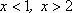inequality notation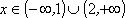interval, or set, notation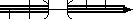number line with parentheses (brackets are used for closed intervals)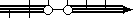number line with open dots (closed dots are used for closed intervals)

2) Factor method. Factoring, I get y = x2  3x + 2 = (x  2)(x  1). Now I will consider each of these factors separately.

The factor x  1 is positive for x > 1; similarly, x  2 is positive for x > 2. Thinking back to when I first learned about negative numbers, I know that (plus)×(plus) = (plus), (minus)×(minus) = (plus), and (minus)×(plus) = (minus). So, to compute the sign on y = x2  3x + 2, I only really need to know the signs on the factors. Then I can apply what I know about multiplying negatives.

 First, I set up a grid, showing the factors and the number line.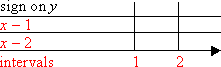Now I mark the intervals where each factor is positive.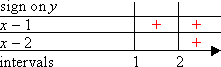Where the factors aren't positive, they must be negative.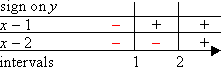Now I multiply up the columns, to compute the sign of y on each interval.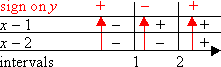Then the solution of x2  3x + 2 > 0 are the two intervals with the "plus" signs:

(negative infinity, 1) and (2, positive infinity).

• Solve 2x2 + 5x + 12 < 0.

First I find the zeroes, which are the endpoints of the intervals: y = 2x2 + 5x + 12 =
(2x  3)(x  4) = 0
for x = 3/2 and x = 4. So the endpoints of the intervals will be at 3/2 and 4. The intervals are between the endpoints, so the intervals are (negative infinity, 3/2], [3/2, 4], and [4, positive infinity). (Note that I use brackets for the endpoints in "or equal to" inequalities, instead of parentheses, because the endpoints will be included in the final solution.)

To find the intervals where y is negative by the Test-Point Method, I just pick a point in each interval. I can use points such as x = 2, x = 0, and x = 5.

To find the intervals where y is negative by the Factor Method, I just solve each factor: 2x  3 is positive for 2x  3 > 0, 3 > 2x, 3/2 > x, or x < 3/2; and x  4 is positive for x  4 > 0,
x > 4
. Then I fill out the grid: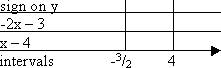Then the solution to this inequality is all x's in

(negative infinity, 3/2 ] and [4, positive infinity).

<< Previous  Top  |  1 | 2 | 3  |  Return to Index  Next >>

 Cite this article as: Stapel, Elizabeth. "Solving Inequalities: An Overview." Purplemath. Available from     https://www.purplemath.com/modules/ineqsolv2.htm. Accessed [Date] [Month] 2016

Reviews of
Internet Sites:
Free Help
Practice
Et Cetera

Study Skills Survey

Tutoring from Purplemath
Find a local math tutor